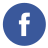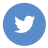### java binary search

java

In Java, the binary search algorithm is a search algorithm that allows you to efficiently search for a specific element in a sorted list of elements. The binary search algorithm works by repeatedly dividing the list in half and comparing the target element with the element in the middle of the list. If the target element is smaller than the middle element, the algorithm searches the left half of the list, and if it is larger, it searches the right half. This process is repeated until the target element is found or it is determined that the element is not in the list.

Here is an example of how to implement a binary search function in Java:

``````import java.util.Arrays;

public class Main {
public static int binarySearch(int[] array, int target) {
// Set the start and end indices of the search range
int start = 0;
int end = array.length - 1;

// Loop until the start index is greater than the end index
while (start <= end) {
// Calculate the middle index of the search range
int mid = (start + end) / 2;

// Compare the target element with the element at the middle index
if (array[mid] == target) {
// The element was found, return the index
return mid;
} else if (array[mid] < target) {
// The target element is larger than the element at the middle index, search the right half of the array
start = mid + 1;
} else {
// The target element is smaller than the element at the middle index, search the left half of the array
end = mid - 1;
}
}

return -1;
}

public static void main(String[] args) {
// Create an array of integers
int[] array = {1, 2, 3, 4, 5, 6, 7, 8, 9, 10};

// Sort the array
Arrays.sort(array);

// Search for the element 5 in the array
int index = binarySearch(array, 5);

// Print the result
System.out.println("The element 5 is at index " + index);
}
}``````

In Java, the `Arrays.binarySearch()` method is used to perform a binary search on an array of elements. A binary search is an efficient algorithm that searches for a specific element in a sorted array by repeatedly dividing the array in half and comparing the element to the middle element of the array.

Here is an example of how to use the `Arrays.binarySearch()` method to search for an element in an array in Java:

``````import java.util.Arrays;

public class Main {
public static void main(String[] args) {
// Create an array of integers
int[] numbers = { 1, 2, 3, 4, 5 };

// Perform a binary search for the number 3
int index = Arrays.binarySearch(numbers, 3);

// Print the index of the element
System.out.println(index); // prints 2
}
}``````

This code creates an array of integers and searches for the number 3 using the `Arrays.binarySearch()` method. The method returns the index of the element, which is 2 in this case.

Keep in mind that the `Arrays.binarySearch()` method only works on sorted arrays, and it returns a negative value if the element is not found in the array.

For more information on the `Arrays.binarySearch()` method and how to use it in Java, you can refer to the Java documentation: